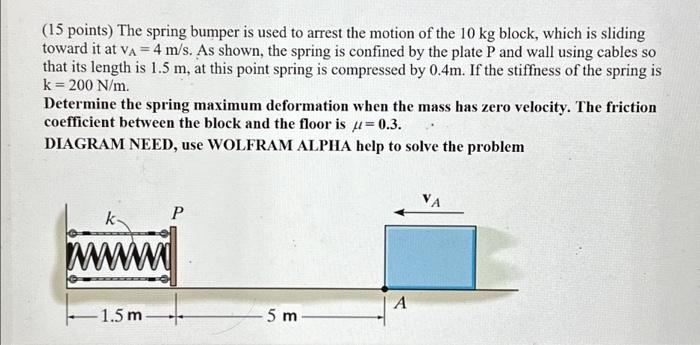Home / Expert Answers / Mechanical Engineering / the-spring-bumper-is-used-to-arrest-the-motion-of-the-10-kg-block-which-is-sliding-toward-it-at-va-pa853

# (Solved): The spring bumper is used to arrest the motion of the 10 kg block, which is sliding toward it at VA ...

The spring bumper is used to arrest the motion of the 10 kg block, which is sliding toward it at VA = 4 m/s. As shown, the spring is confined by the plate P and wall using cables so that its length is 1.5 m, at this point spring is compressed by 0.4m. If the stiffness of the spring k = 200 N/m. Determine the spring maximum deformation when the mass has zero velocity. The friction coefficient between the block and the floor is = 0.3. DIAGRAM NEED, use WOLFRAM ALPHA help to solve the problem k www. P 1.5 m 5 m A VA(15 points) The spring bumper is used to arrest the motion of the block, which is sliding toward it at . As shown, the spring is confined by the plate and wall using cables so that its length is , at this point spring is compressed by . If the stiffness of the spring is . Determine the spring maximum deformation when the mass has zero velocity. The friction coefficient between the block and the floor is . DIAGRAM NEED, use WOLFRAM ALPHA help to solve the problem

We have an Answer from Expert